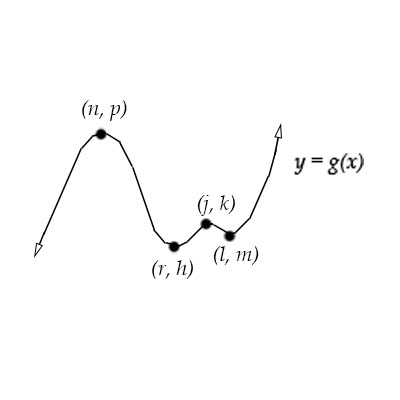# a. Use the extrema to determine the minimum degree of g . b. Give all local extreme points of g...

## Question:

a. Use the extrema to determine the minimum degree of {eq}g {/eq}.

b. Give all local extreme points of {eq}g {/eq}. Tell whether each is a maximum or minimum,

c. Describe all absolute extreme points of {eq}g {/eq},

d. Give the local and absolute extreme values of {eq}g {/eq}.## Extreme Values of Functions

Graphically, absolute maxima and minima points are identified as those points at which the function attain an absolute maximum and minimum values, respectively.

A local maximum/minimum point is a point where the function attain an extreme value and there is an open interval that contains the '{eq}\displaystyle x- {/eq}coordinate of the extreme point.

Therefore, a local extreme cannot be an end-point of the domain, because there is no open interval to contain it and be still part of the domain.

For a point to be a local or an absolute extreme, they should be in the domain of the function.

Graphically, a point is in the domain if is on the continuous line of the graph or at the end of the line but shown with a filled circle.

If the values of a function go to infinity and negative infinity, then there is no absolute extreme point for the function.

a. Knowing that a polynomial function degree {eq}\displaystyle n, {/eq} has at most {eq}\displaystyle n-1 {/eq} turning points,

and because the graph of {eq}\displaystyle g(x) {/eq} in the picture has 4 turning points {eq}\displaystyle (n,p), (r,h), (j,k), \text{ and } (l,m) {/eq}

and the end behavior of the function is like an odd degree, {eq}\displaystyle \boxed{\text{the minimum degree of }g(x) \text{ is }5}. {/eq}

b. The local extreme point of the function, are the point where the {eq}\displaystyle y- {/eq} coordinates are maximum or minimum within the neighborhood of the point.

Meaning that, we can find an open interval containing the point, where the function is wither minimum or maximum, in that interval.

So, the {eq}\displaystyle \boxed{\text{ local extreme points are } x=n, x=r, x=j \text{ and } x=l, \text{ where } x=n, x=j \text{ are local maximum and } x=r \text{ and } x=l\text{ are local minimum points} }. {/eq}

c. The absolute extreme points, are the points where the {eq}\displaystyle y- {/eq} coordinate is the absolute maximum or minimum,.

Because the function goes to infinity for large values of {eq}\displaystyle x {/eq} and negative infinity for negative large values of {eq}\displaystyle x, {/eq} {eq}\displaystyle \boxed{\text{there are no absolute extreme points of the function}}. {/eq}

d. The local extreme values are the {eq}\displaystyle y- {/eq} coordinates of the local extreme points, so {eq}\displaystyle \boxed{\text{ the local extreme values are } y=p, y=h, y=k, \text{ and } y=m}. {/eq}

Because there are no absolute extreme points, {eq}\displaystyle \boxed{\text{ there are no absolute values of the function}}. {/eq}